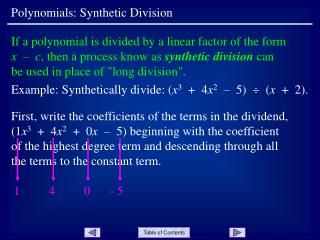DownloadDownload PresentationPolynomials: Synthetic Division

# Polynomials: Synthetic Division

Télécharger la présentation## Polynomials: Synthetic Division

- - - - - - - - - - - - - - - - - - - - - - - - - - - E N D - - - - - - - - - - - - - - - - - - - - - - - - - - -
##### Presentation Transcript

1. 1 4 0 - 5 Polynomials: Synthetic Division If a polynomial is divided by a linear factor of the form x – c, then a process know as synthetic division can be used in place of "long division". Example: Synthetically divide: (x3 + 4x2 – 5)  (x + 2). First, write the coefficients of the terms in the dividend, (1x3 + 4x2 + 0x – 5) beginning with the coefficient of the highest degree term and descending through all the terms to the constant term.

2. Next, write the value of cin the position shown along with the line segments. Note, the divisor is (x + 2). Compare with x – c to see that c is - 2. + + + - 2 - 4 8 1 - 2 2 3 - 4 multiply Polynomials: Synthetic Division 1 4 0 - 5 Next, write the first entry in the dividend row as shown. Next, multiply this value by c and place the result in the next column as shown. Add the column figures as shown. Repeat this process of multiplying and adding as shown Slide 2

3. 1 4 0 - 5 - 2 - 4 8 1 - 2 2 - 4 3 1x2 + 2x – 4 REM QUOTIENT Polynomials: Synthetic Division Last, write the quotient polynomial and remainder by interpreting the row of numbers below the line as shown. Note the degree of the quotient is one less than the degree of the divisor. Try: Synthetically divide: (2x3 – 30x + 1)  (x – 4). The quotient is 2x2 + 8x + 2 and the remainder is 9. Slide 3

4. Polynomials: Synthetic Division END OF PRESENTATION Click to rerun the slideshow.